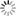# Episode List

OR

### Season 6

27 Sep. 1956
The Big CatBe the first one to add a plot.

11 Oct. 1956
The Big MissusBe the first one to add a plot.

18 Oct. 1956
The Big BeerBe the first one to add a plot.

25 Oct. 1956
The Big Net
9.0 (11)Friday and Smith attempt to stop a robber accused of assaulting and robbing women.

1 Nov. 1956
The Big ConvertibleBe the first one to add a plot.

8 Nov. 1956
The Big LimpBe the first one to add a plot.

15 Nov. 1956
The Big TattooBe the first one to add a plot.

22 Nov. 1956
The Big OddBe the first one to add a plot.

29 Nov. 1956
The Big BillBe the first one to add a plot.

6 Dec. 1956
The Big SearchBe the first one to add a plot.

13 Dec. 1956
The Big TalkBe the first one to add a plot.

20 Dec. 1956
The Big Doting MotherBe the first one to add a plot.

27 Dec. 1956
The Big HandcuffsBe the first one to add a plot.

3 Jan. 1957
The Big FatherBe the first one to add a plot.

10 Jan. 1957
The Big Switch
8.0A suicide attempt leaves a woman in a coma. Friday, Smith need to find the reason.

17 Jan. 1957
The Big StealBe the first one to add a plot.

24 Jan. 1957
The Big ManikinBe the first one to add a plot.

31 Jan. 1957
The Big No ToothBe the first one to add a plot.

7 Feb. 1957
The Big SkipThe Big Skip: A man is reported missing, by two different women, the description matches, but the names are different. He is married to these two and has apparently skipped out on both. Friday searches for him at various places.

14 Feb. 1957
The Big FinBe the first one to add a plot.

21 Feb. 1957
The Big Game
7.6Be the first one to add a plot.

28 Feb. 1957
The Big CoinsBe the first one to add a plot.

7 Mar. 1957
The Big CloseBe the first one to add a plot.

14 Mar. 1957
The Big TieBe the first one to add a plot.

21 Mar. 1957
The Big CupBe the first one to add a plot.

28 Mar. 1957
The Big TruckBe the first one to add a plot.

4 Apr. 1957
The Big SaintBe the first one to add a plot.

11 Apr. 1957
The Big MatchBe the first one to add a plot.

18 Apr. 1957
The Big HelpBe the first one to add a plot.

25 Apr. 1957
The Big CelebrationBe the first one to add a plot.

2 May 1957
The Big Cry BabyBe the first one to add a plot.

9 May 1957
The Big Lesson
7.0Be the first one to add a plot.

16 May 1957
The Big SoldierBe the first one to add a plot.

23 May 1957
The Big Make